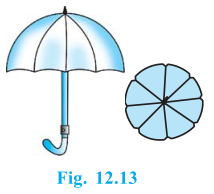# An umbrella has 8 ribs which are equally spaced (see Fig.) . Assuming umbrella to be a flat circle of radius 45 cm, find the area between the two consecutive ribs of the umbrella.Total ribs in umbrella=8

The radius of the umbrella is =45cm

The area between the two consecutive ribs=1/8π*r2

So, the area of the circle (A) = πr2 = (22/7)×(45)=6364.29 cm2

The area between the two consecutive ribs of the umbrella = A/n

6364.29/8 cm2

Or, The area between the two consecutive ribs of the umbrella = 795.5 cm2